Change of basis

Recall: vectors are (1,0) tensors, covectors are (0,1) tensors, bilinear forms, such as scalar products, are (0,2) tensors. The determinant function of n vectors over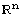, equivalently a n×n matrix, is a (0,n) tensor. Matrices are (1,1) tensors. The commutator table of a Lie algebra is denoted by ad, which is a (1,2) tensor. The Riemannian curvature is a (1,3)-tensor, which is metrical equivalent to a (0,4)-tensor.

How can we stress the importance of the function Τα, which basis transform a (r,s)-tensor X with respect to the basis-transformation α. Note, α is the matrix that encodes the change of basis, equivalently α is vector space isomorphism. So how can we stress the importance? Lets show the impressive implementation :-)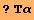Global`Τα

 Τα[X_,r_Integer,α_]:=S[Fold[T[#1,RotateRight[Range[r[X]],1]].#2&,X,Join@@{Table[T[Inv[α]],{r}],Table[α,{r[X]-r}]}]]

The input is tensor X, with contravariant rank r, and basis tranformation α. For example let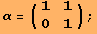We transorm a vector, and a covector by α.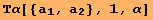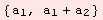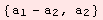For a matrix, we have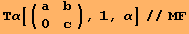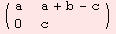For instance in the chapter on Lie algebras, the function Τα is used to transform a given commutator tensor with respect to a change of vector space basis. Furthermore, Τα is the universal tool to extend tensors to left-invariant tensor fields. Briefly: Τα is omnipresent! Another application of Τα is computing the bundle metric. Lets make an easy illustration. Consider the following scalar product defined by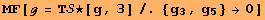Usually, for two vectors U,V, we use notation such as g.U.V of V.g.U in order to compute the scalar-product.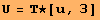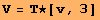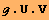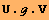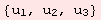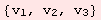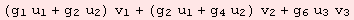A more complicated, but more general formula involves Τα and the information that U,V are (1,0)-tensors.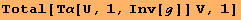For matrices there is the well known scalar product A:B (by notation with respect to g=Id), which yields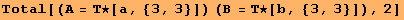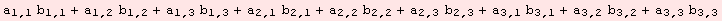Since a matrix is a (1,1)-tensor, we have the equivalent computation by,However, with respect to our special scalar product g as defined above, we would write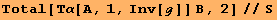The formula is called bundle metric, which is implemented by the command Μg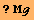Global`Μg

 Μg[g_,X_,Y_,r_Integer:1]:=Total[Τα[X,r,Inv[g]] Y,r[X]]

Application is evident from the discussion above: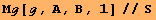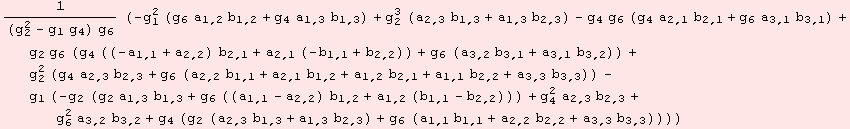The bundle metric easily extends to a norm on the space of (r,s)-tensors.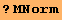Global`ΜNormCreated by Mathematica  (September 30, 2006)